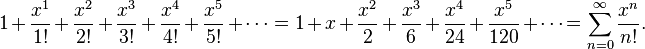# Take care, it’s Floating Point Arithmetic

Yesterday night, I was just idling on IRC and happened to come by an interesting discussion that was taking place there. The original issue was that doing Taylor approximation used to give identity for very small numbers, which gradually turned out to be an enlightening discussion. Thanks to Boris Zbarsky for clearing my doubts on this topic.

This post is a note to self (and others as well) so that I can keep them in mind in future.

For example we want to calculate$e^x - 1$. By the normal Taylor Series expansion of$e^x$, we would get:But if we want to add them in a computer as$x + \frac{x^2}{2!} + \frac{x^3}{3!} + ...$ then you would probably get a correct answer. But if you were to do the same for a very small$x$ you would probably end up getting$x$, which is not correct.

The correct way out of the problem would be to use something like$\frac{x^3}{3!} + \frac{x^2}{2!} + x$. The reason being for a very small$x$ the correct answer is “basically$x$“. So for this we would need to use:

• Next term error estimation to decide how many terms we would want
• Add the terms from smallest to largest

This gives as some important insight into floating point arithmetic. For example we just want to add the following floats$1$, and$2^{25}$ instances of$2^{-25}$. So what is$1 + 2^{-25}$?

You would say almost$1$ (even I thought so). But to the computer that is exactly one. This where floating point arithmetic turns evil, because single precision floats only have$23$ mantissa bits. So the rest of the thing is rounded off, and you get one.

But if you start off by adding with the smaller guys, they can actually accumulate and give the correct result. So if we add$2^{-25}$,$2^{25}$ times and then add$1$, we would get$2$, quite opposite to the previous result.

Floating point arithmetic is not arithmetic we learnt in school. Its not commutative nor associative nor distributive. It also implies that just because these properties are not satisfied, compilers cannot optimize floating point operations either. The same reason why you have -ffast-math to tell gcc just to do your floating point operations fast and not care about the correct answer at all.

Comments on Hacker News.

Advertisements

## 4 thoughts on “Take care, it’s Floating Point Arithmetic”

1.leek says:

Actually, FP addition and multiplication are commutative, just neither associative nor distributive.

The issue in the example is that of cancellation: There are only N bits of precision in a FP number, but when you subtract nearly equal values, like e^x-1 when x is near 0, you cancel out the more significant bits and are left with fewer significant bits than when you started. The bits which get shifted left into the bottom bits of the fixed-precision FP number are not significant, i.e., not accurate. Multiplication and division do not suffer from this cancellation problem that addition and subtraction do, because, except for rounding errors in the bottom bits, multiplication and division retain all of the bits of significance. So if you can rewrite the problem as one of multiplication, such as calculating e^x-1 = x * (series expansion of (e^x-1)/x), you will not lose as many bits of significance in your answer.

2.Mark Liu says:

Your series expansion formula is not correct for e^x – 1, (try substituting x = 0)

1.Sankha Narayan Guria says:

Actually I meant the expansion for e^x only. But I guess it was ambiguous. Anyways I corrected it. 🙂

3.Brad says:

You can use Rational arithmetic libraries in many languages